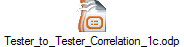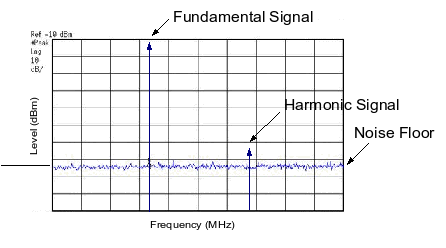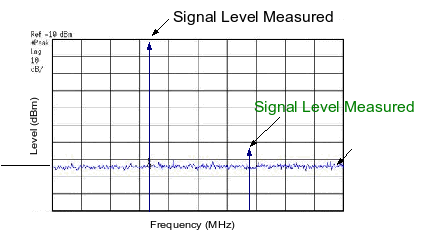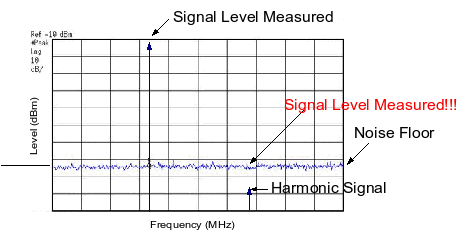Tester to Tester Correlation

Welcome to Roos Instruments discussion of tester to tester correlation of RF measurements. We will be discussing tester accuracy vs tester repeatability, high level vs low level RF measurements and how to correlate both high level and low level measurements. We will also discuss some of the special concerns which relate to performing low level RF measurement correlation and how to avoid these concerns.

Accuracy Vs. Precision (Repeatability)

Accuracy: Degree of Conformity
Precision: Degree of ReproducibilityHigh Accuracy with Low Precision Low Accuracy with High Precision

To start we need to discuss a couple of key terms (and their definitions) which are often used when performing measurement correlation. We also need to understand how these key terms relate to and differ from each other. The key terms we are referring to are: Accuracy and Precision (or Repeatability).
Accuracy is the degree of conformity of a measured or calculated quantity to its actual (true) value.
Precision (or Repeatability) is the degree to which further measurements or calculations will show the same or similar results.

Let's look at the targets shown on this slide to get a better understanding of these definitions and the differences between Accuracy and Precision (Repeatability).
The target to the left shows four hypothetical arrow holes in a target. The holes show good accuracy and poor repeatability. The holes are centered around the center of the target, but are not close to the center. We would say that the mean value of the 4 holes is close to the center of the target even though the holes are not close to the center of the target.
The target to the right shows four different arrow holes in the target. The holes show poor accuracy and good repeatability. The holes are not centered around the center of the target, but the holes are very close together. The mean value of the 4 holes would be to the left and down from the center of the target. We would say that the 4 holes have an offset in both the x and y axis and the standard deviation of the four holes is small compared to the target to the right.

High Level vs Low Level RF Measurements

High Level Measurements

Examples: Output Power, Gain, Gain Flatness

Large Signal levels compared to Measurement Noise Floor

Excellent Measurement Accuracy and Repeatability

Low Level Measurements

Examples: IP3, Harmonics, Phase Noise

Large Measurement Dynamic Range Required

Signal Levels close to the Measurement Noise Floor

Reduced Measurement Accuracy and Repeatability

Now that we agree on Accuracy and Repeatability, let's talk about the differences between high level vs low level RF measurements.

Examples of high level RF measurements are: Output Power, Gain and Gain Flatness.
These measurements typically involve applying relatively large input signals (-30 dBm to 0 dBm) to the device-under- test (DUT) and measuring relatively large output signals from the DUT (-50 dBm to +30 dBm). The accuracy and the repeatability of these measurements are very good, typically better than +0.2 dB accuracy and less than 0.1 dB standard deviation for repeatability.

Examples of low level RF measurements include: IP3 (Third order Intermodulation Distortion), Harmonics and Phase Noise.
These measurements typically involve measuring very low level signals in the presence of high level signals. For example a harmonic measurement may require measuring a -55 dBm harmonic signal in the presence of a +18 dBm fundamental signal. Thus the difference in the signal levels would be +73 dB (+18 dBm - - 55 dBm = +73dB.) A large measurement dynamic range is required to accurately measure both signals. The measurement noise floor will many times become the limiting factor for making the measurement accurately. The accuracy and the repeatability of these measurements are degraded compared to the high level measurements, typically +1 dB accuracy and 0.5 dB standard deviation for repeatability.

Correlation of High Level Measurements

Compare Test Results and Compute Offset

Gain Measurement Example:

Test the Golden Part using Tester A and Tester B

Tester A

Pin = -20.0 dBm, Pout = +5.4 dBm,

Gain = Pout – Pin = +25.4 dB

Tester B:

Pin = -20.0 dBm, Pout = +5.0 dB

Gain = Pout – Pin = +25.0 dB.

Gain Offset = A vs B = 25.4 dB – 25.0 dB = 0.4 dB

Correlating two testers for high level measurements is typically done by comparing the values measured by the two testers and applying a fixed offset. For the example shown we have tester A and tester B. Tester A measures +25.4 dB of Gain and Tester B measures +25 dB of gain for the same golden part. The measurement gain offset between the testers is the difference of these two values which is equal to 0.4 dB. Typical gain offsets vary from 0 to +0.5 dB. Gain offsets greater than +1 dB indicate setup problems which should be investigated.

Correlation of Low Level Measurements

More Complex than High Level Measurements

Devices under Test (DUTs) have Very High Linearity

Fundamental Signal Level Measured is Very Large

Intermodulation & Harmonic Signal Levels are Very Small

Are You Measuring the Device or the Measurement Noise Floor?

Correlating low level measurements is a more complex process. The low level tests are used to measure the linearity of state-of-the-art devices with exceptional linearity performance and these measurements often stress the dynamic range performance of the tester. The question you need to ask and you need to verify is: “Are you measuring the device or the tester's performance?” This is common problem that test engineers understand. The difficulty with low level RF measurements is you may be able to measure the high level signal accurately, however are you measuring the low level signal or the measurement noise floor?

Measurement Noise Floor

Measurement Noise Floor Contributors

Device Noise

Tester Noise

Test Environment Noise Contributors

RF Interference - Radiated Signal and Noise Pickup

Signal Path Leakage

We need to recognize that there are several signal and noise sources in any test environment which will contribute to the measurement noise floor. Each of these noise and signal contributors should be understood, measured and minimized if possible. Remember that the largest contributor will vary from test condition to test condition. So don't assume everything is OK until you verify the results.

Measuring Low Level Signals or the Noise Floor?It is now time to make the low level measurements. The examples shown below are a second harmonic test with two different test conditions.

2nd Harmonic Above Noise FloorIn the plot above the low level second harmonic signal is approximately -73 dBm and approximately 10 dB above the noise floor. The high level fundamental signal is approximately -13 dBm. The harmonic performance measured would be 60 dB (-13 dBm - - 73 dBm = +60 dB).

2nd Harmonic Below Noise FloorIn the next example above the low level second harmonic signal is approximately -96 dBm and approximately 12 dB below the noise floor. The high level fundamental signal is again approximately -13 dBm. The actual harmonic performance of the DUT is 83 dB (-13 dBm - - 96 dBm = +83 dB). However the measured harmonic performance would be only +71 dB (-13 dBm - - 84 dBm = +71 dB). The measurement performance is limited by the measurement noise floor (-84 dBm). This is a 12 dB error. Tester to tester correlation is not possible nor reasonable when the signals being measured are below the noise floor of the testers.

Correlation of Low Level Measurements

1) Verify Signal Levels are above Measurement Noise Floor

Increase Input Signal Level by 1 dB

2nd Harmonic Signal Level Should Increase 2 dB

2nd Order Intermodulation Signal Level Should Increase 2 dB

3rd Harmonic Signal Level Should Increase 3 dB

3rd Order Intermodulation Signal Level Should Increase 3 dB

Listed above are some simple measurement techniques to verify that the low level measurements are not below the noise floor. The process is to increase the input power to the DUT in 1 dB steps and to measure the low level harmonic or intermodulation distortion signal. If the low level signal being measured is above the measurement noise floor, the low level signal will increase in amplitude as shown above.

Correlation of Low Level Measurements

2) If Harmonic or Intermodulation Signals Respond Correctly

Compare Test Results and Compute Offset

2nd Harmonic Measurement Example:

Test the Golden Part using Tester A and Tester B

Tester A Set Pin such that Pout = +18 dBm,

Measured 2nd Harmonic = -47.5 dBm

2nd Harmonic (dBc) = +18 --47.5 = +65.5 dB

Tester B: Set Pin such that Pout = +18 dBm,

Measured 2nd Harmonic = -47 dBm

2nd Harmonic (dBc) = +18 --47 = +65.0 dB

2nd Harmonic Offset = A vs B = 65.5 dB – 65.0 dB = 0.5 dB

Once you confirm that the harmonic or intermodulation signal is responding correctly, you are ready to perform the tester to tester correlation. The correlation process would be the same as for the high level measurements.
For the example shown we have tester A and tester B again. Tester A measures +65.5 dB second harmonic performance and Tester B measures +65 dB second harmonic performance for the same golden part. The second harmonic performance offset between the testers is the difference of these two values which is equal to 0.5 dB. Typical harmonic offsets vary from 0 to +2 dB. Harmonic offsets greater than +2 dB indicate setup problems which should be investigated.

Summary and Conclusion

Accuracy vs Precision

High Level vs Low Level Measurements

Low Level Measurements Require Special Care

Verify the Low Level Measurements are above the Measurement Noise Floor Before Performing Correlation

Both Accuracy and Precision are needed to perform tester to tester correlation. When you perform high level measurement correlation you should expect the offsets to be small in comparison with low level measurement correlation. When performing low level measurement correlation verify that the signals measured are at least 10 dB above the noise floor. Use the techniques show in the presentation to verify that the signals are repeatable and above the noise floor.

https://roos.com/docs/RBEH-72ZS9A
ROOS INSTRUMENTS CONFIDENTIAL AND PROPRIETARY